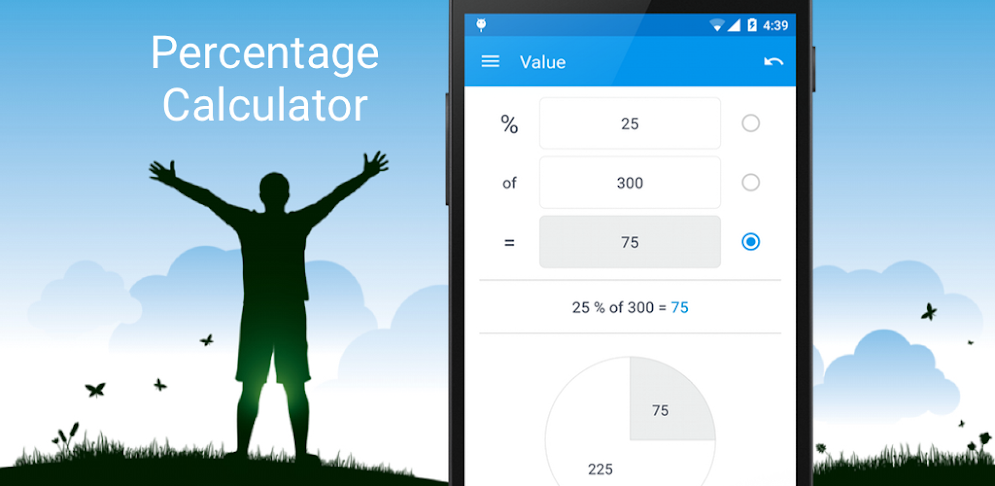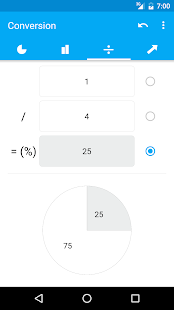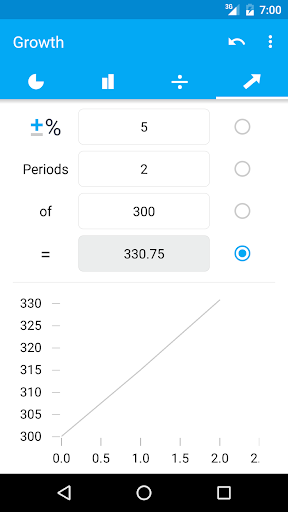# Percentage Calculator## Description

Percentage Calculator is simply the fastest way to calculate discounts, tips, interest and other percentages.

Instantly calculate and visualize all kind of percentages. Any input value can either be a source or result of a calculation — just enter the values you know and it will calculate the remaining one.

The supported calculators and their possible use cases of this Percentage Calculator are:

• Value: (Sales Tax, VAT, School (mathematics, statistics, algebra), Fat Percentage in Food, Cooking Ingredients)
• Change: (Percentage Increase/Decrease, Tips, Markups, Profit Margin, Shopping Discounts, Sale Price, Net/Gross Price; Return on Investment (ROI)
• Conversiom: (Convert Fractions)
• Growth: (Business and Financial, Compound Interest, Inflation, Cumulative Growth, Compound Annual Growth Rate (CAGR), Doubling Time (Rule of 72))

## Screenshots